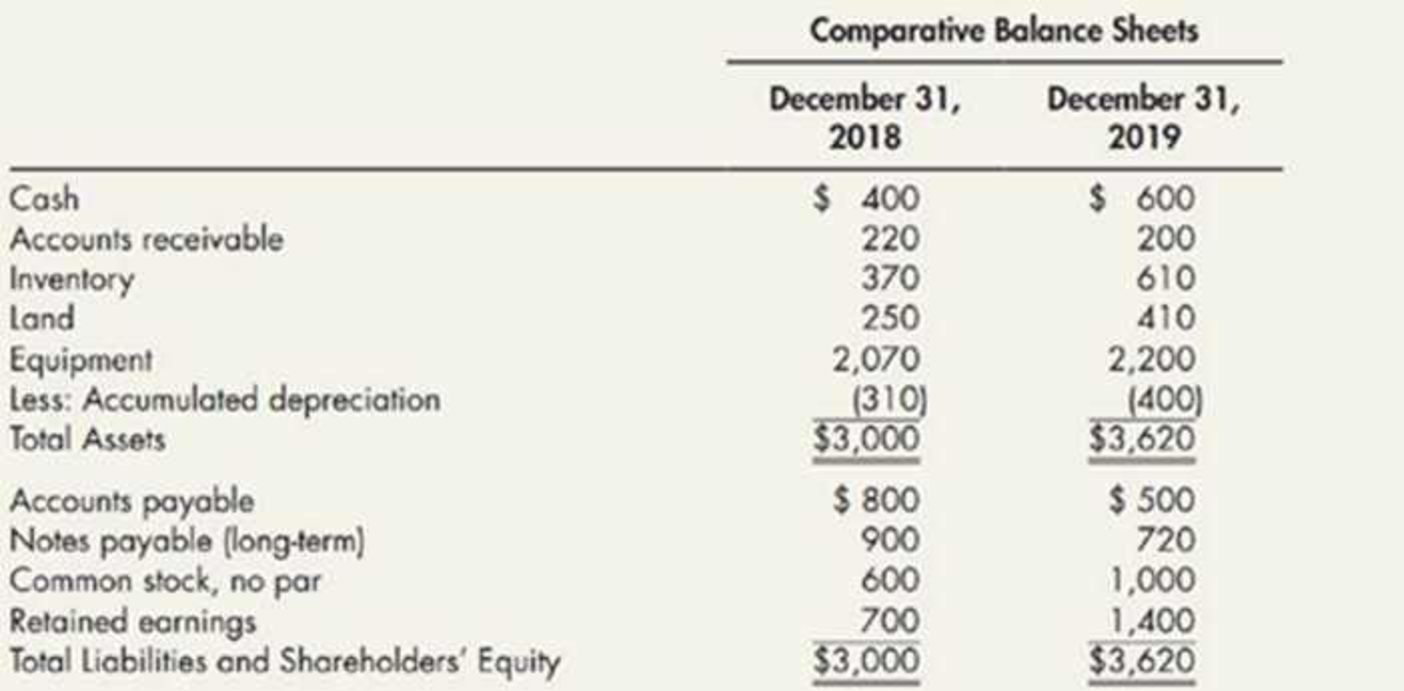Chapter 21, Problem 10E### Intermediate Accounting: Reporting...

3rd Edition
James M. Wahlen + 2 others
ISBN: 9781337788281

#### Solutions

Chapter
Section### Intermediate Accounting: Reporting...

3rd Edition
James M. Wahlen + 2 others
ISBN: 9781337788281
Textbook Problem
5 views

# Spreadsheet The following 2019 information is available for Payne Company:Partial additional information: The net income for 2019 totaled $1,600. During 2019, the company sold, for$390, equipment that cost $390 and had a book value of$300. The company sold land for $200, resulting in a loss of$40. The remaining change in the Land account resulted from the purchase of land through the issuance of common stock.Required:Making whatever additional assumptions that are necessary, prepare a spreadsheet to support the 2019 statement of cash flows for Payne.

To determine

Prepare a statement of cash flows using spreadsheet method of P Company for the year 2019.

Explanation

Statement of cash flows: This statement reports all the cash transactions which are responsible for inflow and outflow of cash, and result of these transactions is reported as ending balance of cash at the end of reported period. Statement of cash flows includes the changes in cash balance due to operating, investing, and financing activities.

Worksheet: A worksheet is a spreadsheet used while preparing a financial statement. It is a type of form having multiple columns and it is used in the adjustment process. The use of a worksheet is optional for any organization. A worksheet can neither be considered as a journal nor a part of the general ledger.

Prepare a statement of cash flows using spreadsheet method.

Table (1)

Table (1)

Working notes:

a. Net income for the year is $1,600 b. Calculate the gain on sale of equipment. Gain on Sale of Equipment=(Cost of equipmentBook value)=$390$300=$90

c. Calculate the loss on sale of land.

Sale of land=(Proceeds from sale of land +Loss on sale of land)=$200+$40=$240 d-1. Calculate the issuance of common stock for land. Issuance of Common Stock=(Beginning year's common stock Ending year's common stock)=$600$1,000=$400

d-2. Calculate the acquisition of land by issuance of common stock.

(Acquisition of land by issuance of common stock)=(Increase in land+Sale of land)=$160+$240=$400 e. Calculate the decrease in accounts receivable. Decrease in Accounts Receivable=(Beginning year's accounts receivable Ending year's accounts receivable)=$220$200=$20

f. Calculate the increase in inventory.

Increase in Inventory=(Beginning year's inventory Ending year's inventory)=$370610=$240

g

### Still sussing out bartleby?

Check out a sample textbook solution.

See a sample solution

#### The Solution to Your Study Problems

Bartleby provides explanations to thousands of textbook problems written by our experts, many with advanced degrees!

Get Started